Tutorial
< Back to the blog

# A Simpson's quick start guide to any Machine Learning image classification project with organized trackable datasets

Getting data ready to train a Machine Learning (ML) model is usually a very time-consuming task and can end up representing half of the time spent on a Machine Learning project. Starting quickly and efficiently is crucial for most projects. This article will help you start your multiclass classification projects in a second!

We all know that real world data is messy. However, without a clean, organized, and easily accessible dataset, Machine Learning projects won’t lead to good results, ever. Try changing every hyperparameter a hundred times, if you don’t have a good dataset, this is a total waste of time and energy.

Therefore, starting any ML projects with an organized structure and the right tools is more than essential. In this article, we will use an easy and efficient way to start a multiclass classification project using the new amazing features from Activeloop Hub - a dataset management tool for deep learning applications (with a focus on computer vision).

### Automatic creation of the dataset with hub.auto

For this example, we will use the Kaggle Simpsons Characters Dataset (if you too, you started to re-watch ALL episodes from the beginning when the pandemic started, and you are now only halfway through all seasons, you will have a lot of fun with this project). This Kaggle dataset gathers jpg images of every character directly taken and labeled from TV show episodes.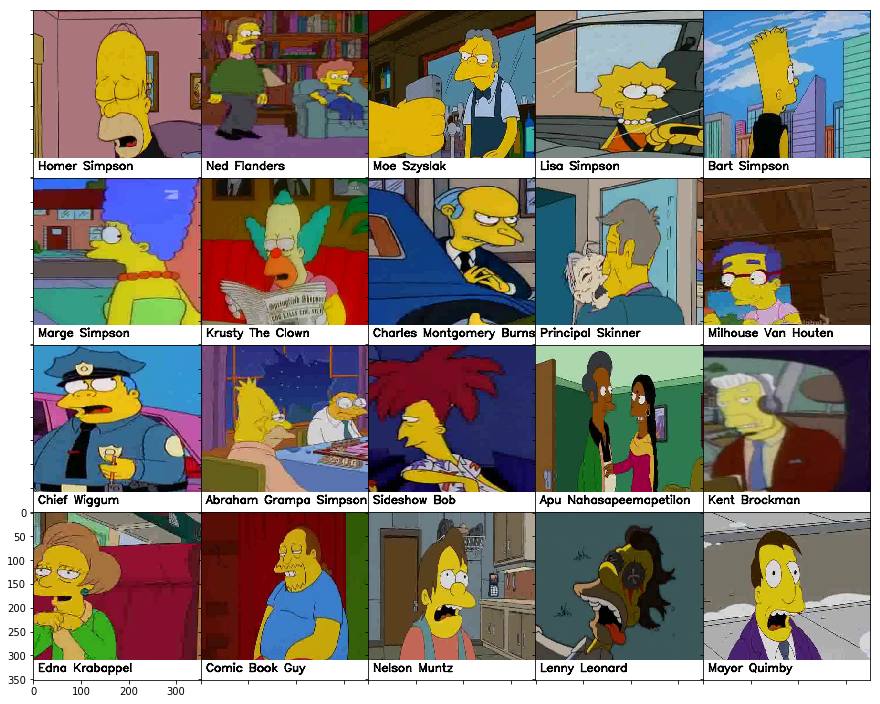It can easily be downloaded using this command line:

``export KAGGLE_USERNAME=”xxxx” && export KAGGLE_KEY=”xxx” && mkdir -p data && cd data && kaggle datasets download -d alexattia/the-simpsons-characters-dataset && unzip -n the-simpsons-characters-dataset.zip``

Now, let’s take a look at the structure of the directory: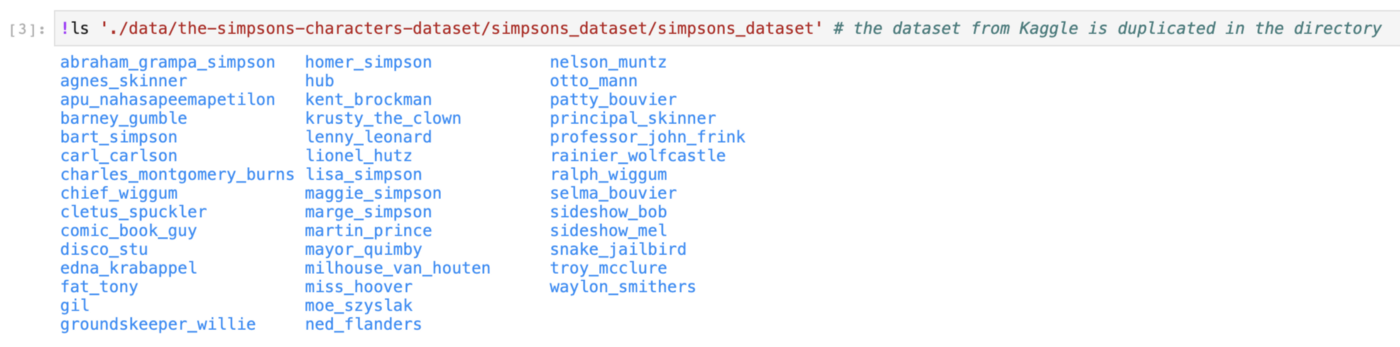We can see that all characters have their own subfolder with their name. Lisa would be so happy.

Once we have the dataset downloaded, we use the Hub feature called Auto Create that will parse the image classification dataset. First, we need to install Hub, if not already done:

``pip install hub==1.3.5``

Then:

``````from hub import Dataset

dataset_path = './data/the-simpsons-characters-dataset/simpsons_dataset/simpsons_dataset'

ds = Dataset.from_path(dataset_path)``````

NB: the variable dataset_path is the path to the dataset’s directory that contains all the images organized in subfolders corresponding to their respective classes — for example, all Lisa Simpson images are in the subfolder “./data/the-simpsons-characters-dataset/simpsons_dataset/lisa_simpson”. The image classification directory needs to be organized like this for the Hub Auto Create feature to be able to work correctly.

We can then take a look at the dataset ds:

``print(ds.shape)``

returns: `(20933,)`. So we know there are 20933 images in the dataset.

``print(ds.schema)``

returns:

``SchemaDict({'image': Image(shape=(None, None, None), dtype='uint8', max_shape=(1072, 1912, 3)), 'label': ClassLabel(shape=(), dtype='uint16', names=['abraham_grampa_simpson', 'agnes_skinner', 'apu_nahasapeemapetilon', 'barney_gumble', 'bart_simpson', 'carl_carlson', 'charles_montgomery_burns', 'chief_wiggum', 'cletus_spuckler', 'comic_book_guy', 'disco_stu', 'edna_krabappel', 'fat_tony', 'gil', 'groundskeeper_willie', 'homer_simpson', 'kent_brockman', 'krusty_the_clown', 'lenny_leonard', 'lionel_hutz', 'lisa_simpson', 'maggie_simpson', 'marge_simpson', 'martin_prince', 'mayor_quimby', 'milhouse_van_houten', 'miss_hoover', 'moe_szyslak', 'ned_flanders', 'nelson_muntz', 'otto_mann', 'patty_bouvier', 'principal_skinner', 'professor_john_frink', 'rainier_wolfcastle', 'ralph_wiggum', 'selma_bouvier', 'sideshow_bob', 'sideshow_mel', 'snake_jailbird', 'troy_mcclure', 'waylon_smithers'], num_classes=42)})``

We see here that there are 42 classes in the dataset ds.

Let’s visualize 6 random images from the dataset:

``````def show_image_in_ds(ds, idx=1):
image = ds[‘image’, idx].compute()
label = ds[‘label’, idx].compute(label_name=True)
print(“Image:”)
plt.imshow(image)
plt.show()
print(“Label: \”%s\”” % (label))

import random
num_images_to_display = 6
for id in range(0,num_images_to_display):
show_image_in_ds(ds, random.randint(0,ds.shape))``````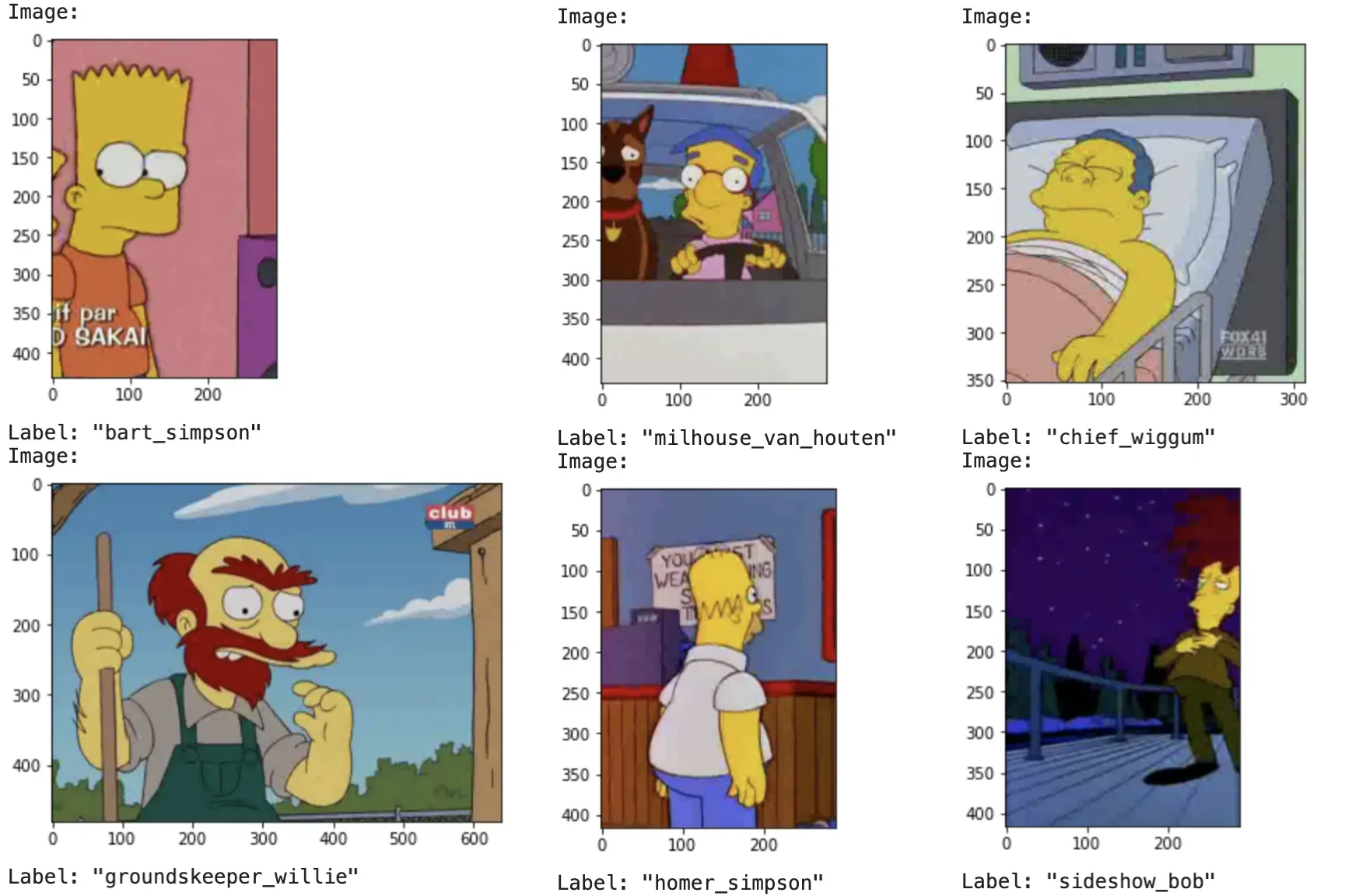As we can see here, the images have different sizes and need to be resized to a common size for training. For this, we can use the feature Hub transform feature:

``````import hub
from skimage.transform import resize
from skimage import img_as_ubyte

# resize images
new_shape = (256, 256, 3)
new_schema = {
"image": schema.Image(shape=new_shape, dtype="uint8"),
"label": schema.ClassLabel(names=['abraham_grampa_simpson', 'agnes_skinner', 'apu_nahasapeemapetilon', 'barney_gumble', 'bart_simpson', 'carl_carlson', 'charles_montgomery_burns', 'chief_wiggum', 'cletus_spuckler', 'comic_book_guy', 'disco_stu', 'edna_krabappel', 'fat_tony', 'gil', 'groundskeeper_willie', 'homer_simpson', 'kent_brockman', 'krusty_the_clown', 'lenny_leonard', 'lionel_hutz', 'lisa_simpson', 'maggie_simpson', 'marge_simpson', 'martin_prince', 'mayor_quimby', 'milhouse_van_houten', 'miss_hoover', 'moe_szyslak', 'ned_flanders', 'nelson_muntz', 'otto_mann', 'patty_bouvier', 'principal_skinner', 'professor_john_frink', 'rainier_wolfcastle', 'ralph_wiggum', 'selma_bouvier', 'sideshow_bob', 'sideshow_mel', 'snake_jailbird', 'troy_mcclure', 'waylon_smithers'])
}

@hub.transform(schema=new_schema)
def resize_transform(index):
image = resize(ds['image', index].compute(), new_shape, anti_aliasing=True)
image = img_as_ubyte(image)  # recast from float to uint8
label = int(ds['label', index].compute())
return {
"image": image,
"label": label
}

ds_r = resize_transform(range(ds.shape))``````

Now we want to store the resized dataset in Hub:

``````url = "margauxmforsythe/simpsons_resized_256x256"
# This will take some time as there are 20k images in the dataset
ds_r.store(url)``````

Then the dataset is available and can be visualized in the Activeloop's visualization app: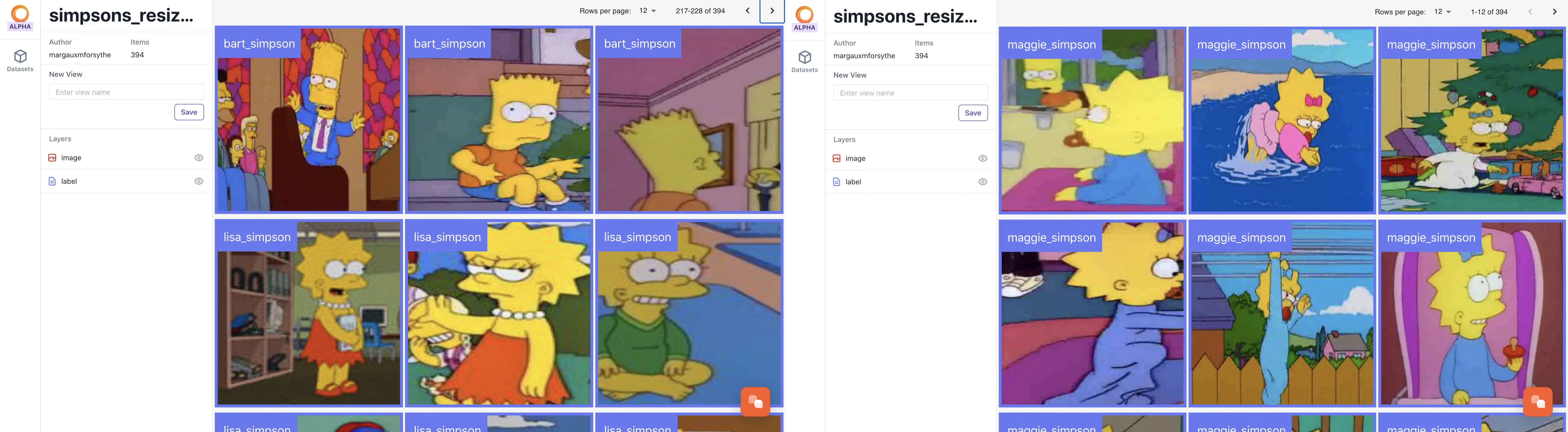or can be loaded using the url:

``````ds_from_hub = Dataset(url)

# Visualize the images and labels
def show_image_in_ds(ds, idx=1):
image = ds['image', idx].compute()
label = ds['label', idx].compute(label_name=True)
print("Image:")
print(image.shape)
plt.imshow(image)
plt.show()
print("Label: \"%s\"" % (label))

for i in range(6):
show_image_in_ds(ds_from_hub, i)``````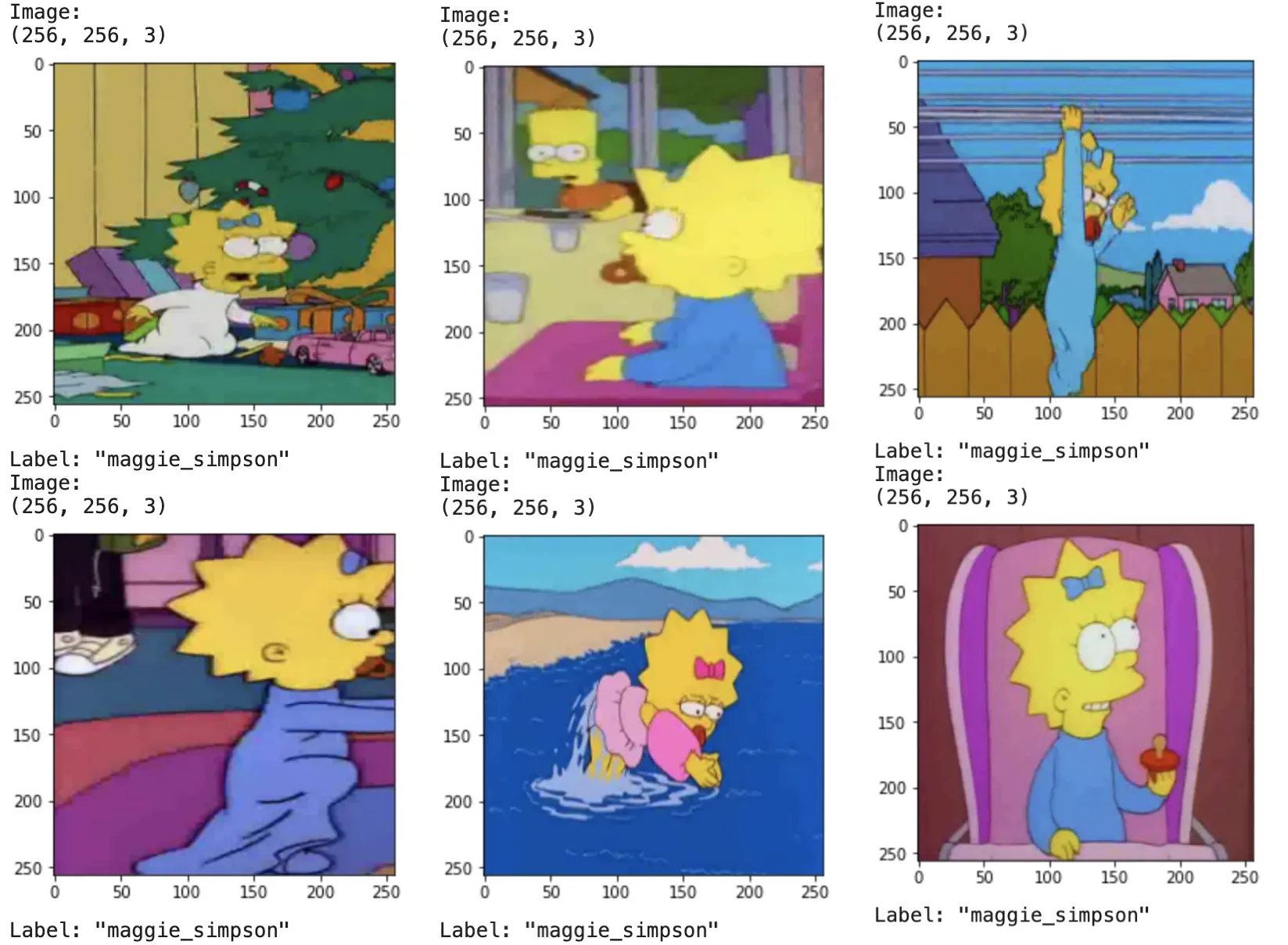### Dataset Filtering / Variants of the same dataset

Using the filter feature of Hub, we can easily create subsets of the dataset or get rid of elements not needed in the training.

#### Create a subset dataset with only some selected characters

##### Maggie

For example, if we want to create a subset of Maggie’s images, we filter the dataset and only keep the items that labels start with “maggie”:

``````# Creates a DatasetView object for a subset of the Dataset.
ds_only_maggie = ds_from_hub.filter(lambda x: x["label"].compute(label_name=True).startswith("maggie"))``````

So here, the filter takes in the dataset ds and return True or False if the label start with “maggie”. Then the function is applied to all the items of the datasetview and retains only the items that return True, that is to say, Maggie’s images.

We can check if the number of images we now have in the subset is correct:

``````number_maggie_images_in_subset = len(ds_only_maggie)
path_to_maggie_images = './data/the-simpsons-characters-dataset/simpsons_dataset/simpsons_dataset/maggie_simpson'
number_maggie_imgs = len(glob(f"{path_to_maggie_images}/*.jpg"))
assert number_maggie_images_in_subset == number_maggie_imgs
print(number_maggie_images_in_subset)``````

which returns: 128. So we know we have 128 images of Maggie in the subset `ds2`.

With the same logic, we can create a subset without Maggie:

``````ds_without_maggie = ds.filter(lambda x: not x["label"].compute(label_name=True).startswith("maggie"))
print(ds.shape - number_maggie_images_in_subset == len(ds_without_maggie)) #shape is (20805,)``````

which returns `True`, so we know that all 128 images of Maggie were removed.

##### A Simpsons' Family Photo (Dataset)

Now we want to create a subset of the Simpsons family only: Maggie, Marge, Lisa, Bart, and Homer:

``````# Creates a DatasetView object for a subset of the Dataset.
ds_simpsons_family = ds_from_hub.filter(lambda x: x["label"].compute(label_name=True).startswith("maggie")
or x["label"].compute(label_name=True).startswith("marge")
or x["label"].compute(label_name=True).startswith("lisa")
or x["label"].compute(label_name=True).startswith("bart")
or x["label"].compute(label_name=True).startswith("homer"))

print(len(ds_simpsons_family)) #returns 6361``````

There are 6361 images of the members of the Simpsons family.

### Monitor your datasets without "D'oh!"-s

“Mom, look, I found something more fun than complaining!” — Lisa Simpson

Datasets are, as we said before, the most important part of a training. So why not treat them as we treat scripts? When a training script is modified, we often want to know what changes were made, so that, if something breaks, we can throw back to the previous version of the script — and this is usually done using git.

So, why not do the exact same thing with the datasets? They are even more important than the training script!

Well, that’s what Hub version control is doing. Here is an example with the different versions of the dataset (subsets) we created previously:

Create a new commit “hello world” in the master branch:

``````ds = Dataset(url)
ds.checkout("master")
a = ds.commit("first commit")``````

Create a new branch called “subsets”:

``````ds.checkout("subsets", create=True)  # creates a new branch
ds.flush()
print(ds.branches) # returns dict_keys(['master', 'subsets'])
ds.log()``````

The `ds.log()` returns:

``````Current Branch: subsets

commit 7d8d6c7f891139dba5c13ea57360b854ac6990d6 (master)
Author: margauxmforsythe
Commit Time:  2021-05-20 20:22:46
Message: "first commit"``````

Showing that we are on the branch “subset” and that there was one commit “hello world” sent to the master branch.

Create a commit with only Maggie’s images in the “subsets” branch:

``````ds.checkout("subsets") # checkout to the subsets branch

# Filter the dataset and only keep Maggie's images
dt = ds.filter(lambda x: x["label"].compute(label_name=True).startswith("maggie"))
dt.commit("Maggie images subset")
ds.log()``````

Now the log shows that we are still on the branch “subsets” but now, another commit “Maggie images subset” has been sent to the “subsets” branch:

``````Current Branch: subsets

commit 1b54aa2185d3f61167737a860f7205e15aeef7b6 (subsets)
Author: margauxmforsythe
Commit Time:  2021-05-20 20:25:04
Message: "Maggie images subset"

commit 7d8d6c7f891139dba5c13ea57360b854ac6990d6 (master)
Author: margauxmforsythe
Commit Time:  2021-05-20 20:22:46
Message: "first commit"``````

Commit subset with the Simpsons family:

``````# Filters the Simspons from the datasetdt = ds.filter(lambda x: x["label"].compute(label_name=True).startswith("maggie")
or x["label"].compute(label_name=True).startswith("marge")
or x["label"].compute(label_name=True).startswith("lisa")
or x["label"].compute(label_name=True).startswith("bart")
or x["label"].compute(label_name=True).startswith("homer"))
c = dt.commit("Simpsons family subset")
ds.log()``````

And now the log shows the three commits:

``````Current Branch: subsets

commit 3cf078659a6499f9e6e8bf163cc6926ab2ab3d37 (subsets)
Author: margauxmforsythe
Commit Time:  2021-05-20 20:34:31
Message: "Simpsons family subset"

commit 1b54aa2185d3f61167737a860f7205e15aeef7b6 (subsets)
Author: margauxmforsythe
Commit Time:  2021-05-20 20:25:04
Message: "Maggie images subset"

commit 7d8d6c7f891139dba5c13ea57360b854ac6990d6 (master)
Author: margauxmforsythe
Commit Time:  2021-05-20 20:22:46
Message: "first commit"``````

And finally, we want to go back to the first commit on master branch:

``ds.checkout(a) # reminder we ran: a = ds.commit("first commit")``

which could also be done with this line using the commit id shown in the log:

``ds.checkout('7d8d6c7f891139dba5c13ea57360b854ac6990d6') # from log``

So now, we have two branches and three commits for the dataset corresponding to the url “margauxmforsythe/simpsons_resized_256x256”.

#### Saving the Simpsons family subset as a separate Dataset

Now if we want to use the subset with only the images of the Simpsons family, we can save the subset we created previously and use it for training — but keep the information that there are 42 classes in the original dataset so that we can train with more characters later:

``````ds_S = ds_simpsons_family.store('margauxmforsythe/simpsons_family')
ds_S``````

which returns:

``Dataset(schema=SchemaDict({'image': Image(shape=(256, 256, 3), dtype='uint8'), 'label': ClassLabel(shape=(), dtype='uint8', names=['abraham_grampa_simpson', 'agnes_skinner', 'apu_nahasapeemapetilon', 'barney_gumble', 'bart_simpson', 'carl_carlson', 'charles_montgomery_burns', 'chief_wiggum', 'cletus_spuckler', 'comic_book_guy', 'disco_stu', 'edna_krabappel', 'fat_tony', 'gil', 'groundskeeper_willie', 'homer_simpson', 'kent_brockman', 'krusty_the_clown', 'lenny_leonard', 'lionel_hutz', 'lisa_simpson', 'maggie_simpson', 'marge_simpson', 'martin_prince', 'mayor_quimby', 'milhouse_van_houten', 'miss_hoover', 'moe_szyslak', 'ned_flanders', 'nelson_muntz', 'otto_mann', 'patty_bouvier', 'principal_skinner', 'professor_john_frink', 'rainier_wolfcastle', 'ralph_wiggum', 'selma_bouvier', 'sideshow_bob', 'sideshow_mel', 'snake_jailbird', 'troy_mcclure', 'waylon_smithers'], num_classes=42)}), url='margauxmforsythe/simpsons_family', shape=(6361,), mode='w')``

And if we check in the web app, we see there are 6361 images.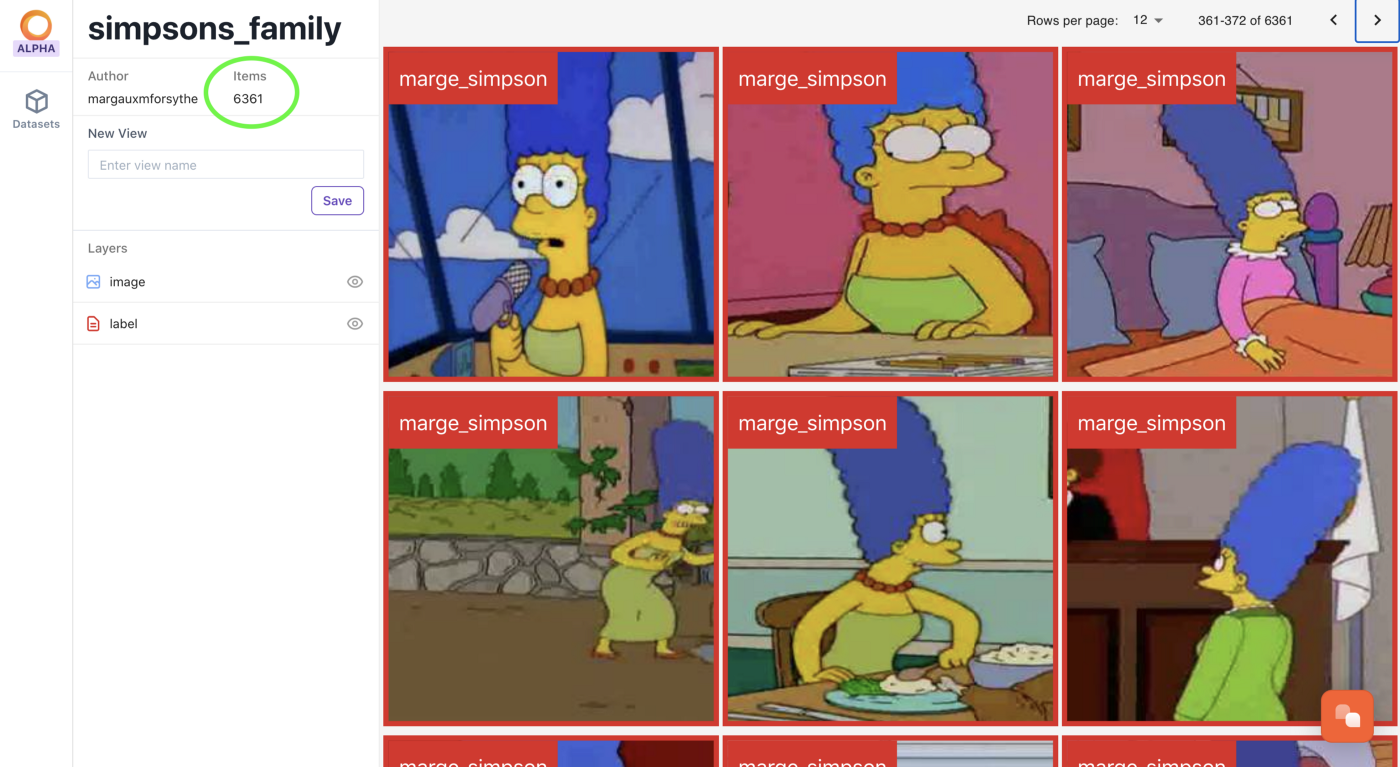Notebook for the dataset manipulation with Hub features here.

#### Now you can manipulate datasets easier than ever before, and start a simple training! Let’s try it!

For the training, we will only use the Simpsons family subset and a simple CNN. The first step is to get the dataset ready for training — we will use Tensorflow and so, will use the Hub feature to_tensorflow:

``````def to_model_fit(item):
x = item["image"]/255 # normalize
y = item["label"]
return (x, y)
image_count = len(ds_S)
print(f"Images count: {image_count}") #Images count: 6361

ds_tf = ds_S.to_tensorflow(include_shapes=True)
ds_tf = ds_tf.map(lambda x: to_model_fit(x))``````

Then we need to shuffle and split the dataset in the train set and validation set with a ratio of 80% of the images used for training, 20% used for validation:

``````train_size = int(0.8 * image_count)
val_size = int(0.2 * image_count)
batch_size = 8
print(f"{train_size} training images and {val_size} validation images. Batch size of {batch_size}")
list_ds = ds_tf.shuffle(image_count)
val_ds = ds_tf.take(val_size)
train_ds = ds_tf.skip(val_size)
train_ds = train_ds.shuffle(train_size)
train_ds = train_ds.batch(batch_size)
val_ds = val_ds.shuffle(val_size)
val_ds = val_ds.batch(batch_size)``````
``=> 5088 training images and 1272 validation images. Batch size of 12``

Now we can define the model, compile it and run the training:

``````model = Simple_CNN_With_Dropout(num_classes)
model.compile(optimizer=tf.keras.optimizers.Adam(0.001),
loss=tf.keras.losses.SparseCategoricalCrossentropy(from_logits=True),metrics=["accuracy"] )
model.fit(train_ds, validation_data=val_ds, epochs = 60)``````

#### Evaluation on the test set

We first need to set up the test set in the same way we set up the previous dataset, by using hub.auto and hub.transform features. We did have to put the test images in subfolders corresponding to their classes beforehand: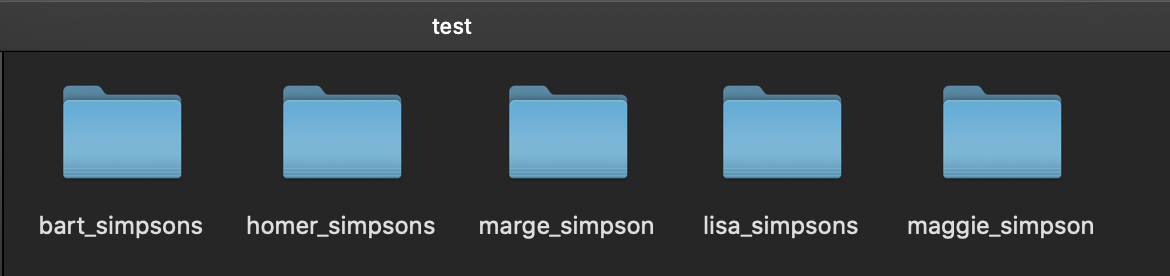``````test_set_path = "./data/the-simpsons-characters-dataset/kaggle_simpson_testset/test"
ds_test = Dataset.from_path(test_set_path)

# resize images
new_shape = (256, 256, 3)
new_schema = {
"image": schema.Image(shape=new_shape, dtype="uint8"),
"label": schema.ClassLabel(names=['bart_simpsons', 'homer_simpsons', 'lisa_simpsons', 'maggie_simpson', 'marge_simpson'])
}

@hub.transform(schema=new_schema)
def resize_transform(index):
image = resize(ds_test['image', index].compute(), new_shape, anti_aliasing=True)
image = img_as_ubyte(image)  # recast from float to uint8
label = int(ds_test['label', index].compute())
return {
"image": image,
"label": label
}
ds_r = resize_transform(range(ds_test.shape))
ds_test = ds_r.store("margauxmforsythe/simpsons_dataset_test")``````

Finally, we ran the model on the test set:

``````ds_test = Dataset("margauxmforsythe/simpsons_dataset_test")
ds_test_pred = ds_test.to_tensorflow(include_shapes=True).batch(1)
ds_tf = ds_test_pred.map(lambda x: to_model_fit(x))
model.predict(ds_tf)
predictions_test_ds = model.predict(ds_tf)
y_pred = []
y_true = []
i = 0

for img, label in ds_tf:
y_true.append(classes_family[label.numpy()])
y_pred.append(classes[np.argmax(predictions_test_ds[i])])
plt.imshow(img)
plt.show()
print(f"Predicted class: {classes[np.argmax(predictions_test_ds[i])]}, real class: {classes_family[label.numpy()]}")
i = i + 1``````

These are some of the results from the predictions on the test set (there was no example of Maggie in the test set):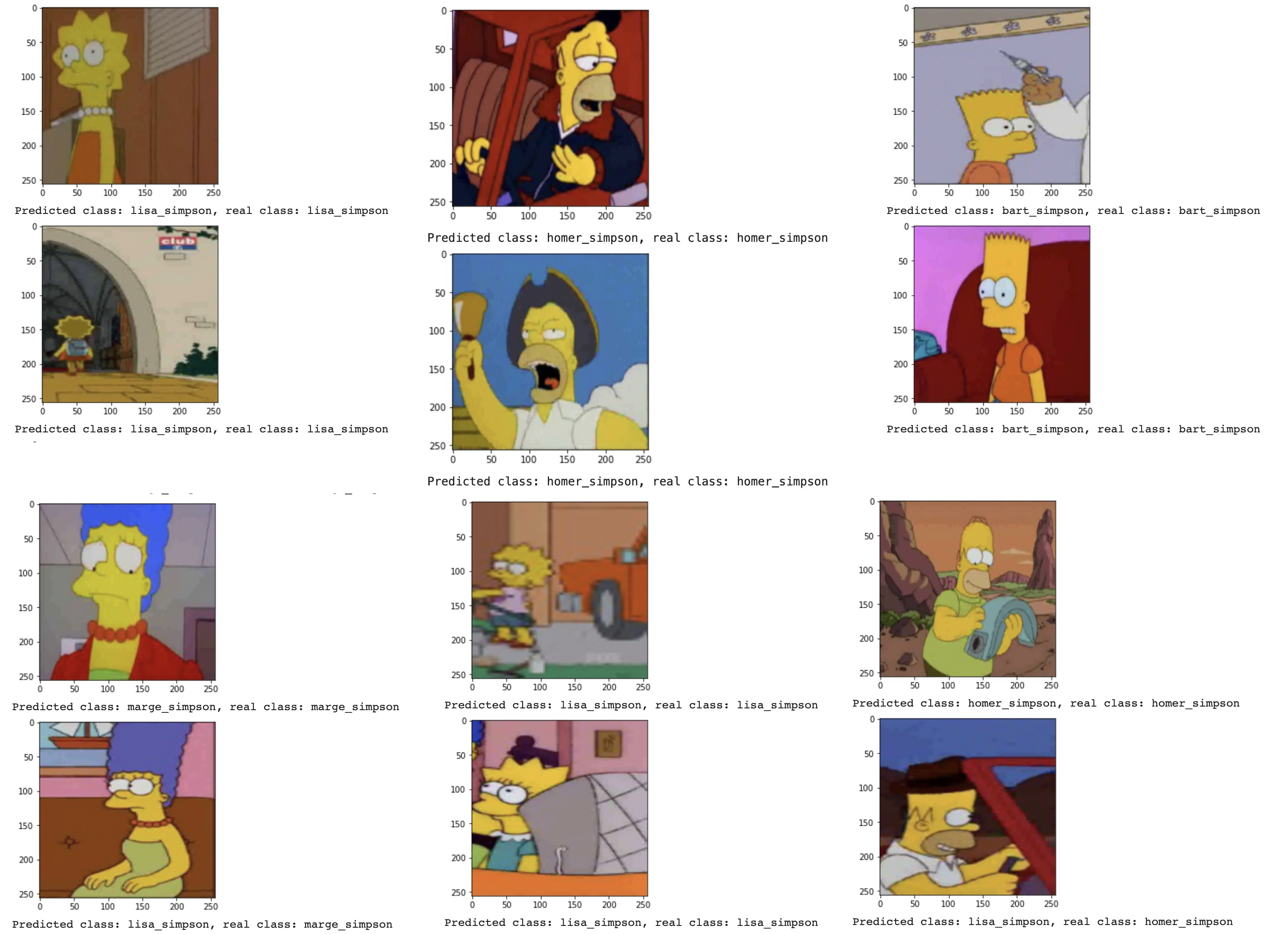The final confusion matrix after 60 epochs:Training notebook using the Hub Datasets is here.

Oh, so they have internet on computers now! — Homer

If you have any questions regarding this tutorial, I'll be at Moe's... ehm, the Hub Slack Community. Feel free to hit us up there - we might even have donuts!

Unifying and abstracting away infrastructure for easier and highly efficient machine and deep learning.